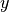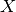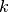Shortcuts

# Classic ML Models¶

This module implements classic machine learning models in PyTorch Lightning, including linear regression and logistic regression. Unlike other libraries that implement these models, here we use PyTorch to enable multi-GPU, multi-TPU and half-precision training.

Note

We rely on the community to keep these updated and working. If something doesn’t work, we’d really appreciate a contribution to fix!

## Linear Regression¶

Linear regression fits a linear model between a real-valued target variableand one or more features. We estimate the regression coefficients that minimize the mean squared error between the predicted and true target values.

We formulate the linear regression model as a single-layer neural network. By default we include only one neuron in the output layer, although you can specify the output_dim yourself.

Add either L1 or L2 regularization, or both, by specifying the regularization strength (default 0).

from pl_bolts.models.regression import LinearRegression
import pytorch_lightning as pl
from pl_bolts.datamodules import SklearnDataModule

model = LinearRegression(input_dim=10)
trainer = pl.Trainer()


Bases: LightningModule

Warning

The feature LinearRegression is currently marked under review. The compatibility with other Lightning projects is not guaranteed and API may change at any time. The API and functionality may change without warning in future releases. More details: https://lightning-bolts.readthedocs.io/en/latest/stability.html

Linear regression model implementing - with optional L1/L2 regularization $$min_{W} ||(Wx + b) - y ||_2^2$$

Parameters:
configure_optimizers()[source]

Choose what optimizers and learning-rate schedulers to use in your optimization. Normally you’d need one. But in the case of GANs or similar you might have multiple.

Return type:

Optimizer

Returns:

Any of these 6 options.

• Single optimizer.

• List or Tuple of optimizers.

• Two lists - The first list has multiple optimizers, and the second has multiple LR schedulers (or multiple lr_scheduler_config).

• Dictionary, with an "optimizer" key, and (optionally) a "lr_scheduler" key whose value is a single LR scheduler or lr_scheduler_config.

• Tuple of dictionaries as described above, with an optional "frequency" key.

• None - Fit will run without any optimizer.

The lr_scheduler_config is a dictionary which contains the scheduler and its associated configuration. The default configuration is shown below.

lr_scheduler_config = {
# REQUIRED: The scheduler instance
"scheduler": lr_scheduler,
# The unit of the scheduler's step size, could also be 'step'.
# 'epoch' updates the scheduler on epoch end whereas 'step'
# updates it after a optimizer update.
"interval": "epoch",
# How many epochs/steps should pass between calls to
# scheduler.step(). 1 corresponds to updating the learning
# rate after every epoch/step.
"frequency": 1,
# Metric to to monitor for schedulers like ReduceLROnPlateau
"monitor": "val_loss",
# If set to True, will enforce that the value specified 'monitor'
# is available when the scheduler is updated, thus stopping
# training if not found. If set to False, it will only produce a warning
"strict": True,
# If using the LearningRateMonitor callback to monitor the
# learning rate progress, this keyword can be used to specify
# a custom logged name
"name": None,
}


When there are schedulers in which the .step() method is conditioned on a value, such as the torch.optim.lr_scheduler.ReduceLROnPlateau scheduler, Lightning requires that the lr_scheduler_config contains the keyword "monitor" set to the metric name that the scheduler should be conditioned on.

# The ReduceLROnPlateau scheduler requires a monitor
def configure_optimizers(self):
return {
"optimizer": optimizer,
"lr_scheduler": {
"scheduler": ReduceLROnPlateau(optimizer, ...),
"monitor": "metric_to_track",
"frequency": "indicates how often the metric is updated"
# If "monitor" references validation metrics, then "frequency" should be set to a
# multiple of "trainer.check_val_every_n_epoch".
},
}

# In the case of two optimizers, only one using the ReduceLROnPlateau scheduler
def configure_optimizers(self):
optimizer2 = SGD(...)
scheduler1 = ReduceLROnPlateau(optimizer1, ...)
scheduler2 = LambdaLR(optimizer2, ...)
return (
{
"optimizer": optimizer1,
"lr_scheduler": {
"scheduler": scheduler1,
"monitor": "metric_to_track",
},
},
{"optimizer": optimizer2, "lr_scheduler": scheduler2},
)


Metrics can be made available to monitor by simply logging it using self.log('metric_to_track', metric_val) in your LightningModule.

Note

The frequency value specified in a dict along with the optimizer key is an int corresponding to the number of sequential batches optimized with the specific optimizer. It should be given to none or to all of the optimizers. There is a difference between passing multiple optimizers in a list, and passing multiple optimizers in dictionaries with a frequency of 1:

• In the former case, all optimizers will operate on the given batch in each optimization step.

• In the latter, only one optimizer will operate on the given batch at every step.

This is different from the frequency value specified in the lr_scheduler_config mentioned above.

def configure_optimizers(self):
optimizer_one = torch.optim.SGD(self.model.parameters(), lr=0.01)
optimizer_two = torch.optim.SGD(self.model.parameters(), lr=0.01)
return [
{"optimizer": optimizer_one, "frequency": 5},
{"optimizer": optimizer_two, "frequency": 10},
]


In this example, the first optimizer will be used for the first 5 steps, the second optimizer for the next 10 steps and that cycle will continue. If an LR scheduler is specified for an optimizer using the lr_scheduler key in the above dict, the scheduler will only be updated when its optimizer is being used.

Examples:

# most cases. no learning rate scheduler
def configure_optimizers(self):

# multiple optimizer case (e.g.: GAN)
def configure_optimizers(self):
return gen_opt, dis_opt

# example with learning rate schedulers
def configure_optimizers(self):
dis_sch = CosineAnnealing(dis_opt, T_max=10)
return [gen_opt, dis_opt], [dis_sch]

# example with step-based learning rate schedulers
# each optimizer has its own scheduler
def configure_optimizers(self):
gen_sch = {
'scheduler': ExponentialLR(gen_opt, 0.99),
'interval': 'step'  # called after each training step
}
dis_sch = CosineAnnealing(dis_opt, T_max=10) # called every epoch
return [gen_opt, dis_opt], [gen_sch, dis_sch]

# example with optimizer frequencies
# see training procedure in Improved Training of Wasserstein GANs, Algorithm 1
# https://arxiv.org/abs/1704.00028
def configure_optimizers(self):
n_critic = 5
return (
{'optimizer': dis_opt, 'frequency': n_critic},
{'optimizer': gen_opt, 'frequency': 1}
)


Note

Some things to know:

• Lightning calls .backward() and .step() on each optimizer as needed.

• If learning rate scheduler is specified in configure_optimizers() with key "interval" (default “epoch”) in the scheduler configuration, Lightning will call the scheduler’s .step() method automatically in case of automatic optimization.

• If you use 16-bit precision (precision=16), Lightning will automatically handle the optimizers.

• If you use multiple optimizers, training_step() will have an additional optimizer_idx parameter.

• If you use torch.optim.LBFGS, Lightning handles the closure function automatically for you.

• If you use multiple optimizers, gradients will be calculated only for the parameters of current optimizer at each training step.

• If you need to control how often those optimizers step or override the default .step() schedule, override the optimizer_step() hook.

forward(x)[source]
Parameters:
• *args – Whatever you decide to pass into the forward method.

• **kwargs – Keyword arguments are also possible.

Return type:

Tensor

Returns:

test_epoch_end(outputs)[source]

Called at the end of a test epoch with the output of all test steps.

# the pseudocode for these calls
test_outs = []
for test_batch in test_data:
out = test_step(test_batch)
test_outs.append(out)
test_epoch_end(test_outs)

Parameters:

outputs (List[Dict[str, Tensor]]) – List of outputs you defined in test_step_end(), or if there are multiple dataloaders, a list containing a list of outputs for each dataloader

Return type:
Returns:

None

Note

If you didn’t define a test_step(), this won’t be called.

Examples

def test_epoch_end(self, outputs):
# do something with the outputs of all test batches
all_test_preds = test_step_outputs.predictions

some_result = calc_all_results(all_test_preds)
self.log(some_result)


With multiple dataloaders, outputs will be a list of lists. The outer list contains one entry per dataloader, while the inner list contains the individual outputs of each test step for that dataloader.

def test_epoch_end(self, outputs):
final_value = 0
# do something
final_value += test_step_out

self.log("final_metric", final_value)

test_step(batch, batch_idx)[source]

Operates on a single batch of data from the test set. In this step you’d normally generate examples or calculate anything of interest such as accuracy.

# the pseudocode for these calls
test_outs = []
for test_batch in test_data:
out = test_step(test_batch)
test_outs.append(out)
test_epoch_end(test_outs)

Parameters:
Return type:
Returns:

Any of.

• Any object or value

• None - Testing will skip to the next batch

# if you have one test dataloader:
def test_step(self, batch, batch_idx):
...

# if you have multiple test dataloaders:
...


Examples:

# CASE 1: A single test dataset
def test_step(self, batch, batch_idx):
x, y = batch

out = self(x)
loss = self.loss(out, y)

# log 6 example images
# or generated text... or whatever
sample_imgs = x[:6]
grid = torchvision.utils.make_grid(sample_imgs)

# calculate acc
labels_hat = torch.argmax(out, dim=1)
test_acc = torch.sum(y == labels_hat).item() / (len(y) * 1.0)

# log the outputs!
self.log_dict({'test_loss': loss, 'test_acc': test_acc})


If you pass in multiple test dataloaders, test_step() will have an additional argument. We recommend setting the default value of 0 so that you can quickly switch between single and multiple dataloaders.

# CASE 2: multiple test dataloaders
# dataloader_idx tells you which dataset this is.
...


Note

If you don’t need to test you don’t need to implement this method.

Note

When the test_step() is called, the model has been put in eval mode and PyTorch gradients have been disabled. At the end of the test epoch, the model goes back to training mode and gradients are enabled.

training_step(batch, batch_idx)[source]

Here you compute and return the training loss and some additional metrics for e.g. the progress bar or logger.

Parameters:
Return type:
Returns:

Any of.

• Tensor - The loss tensor

• dict - A dictionary. Can include any keys, but must include the key 'loss'

• None - Training will skip to the next batch. This is only for automatic optimization.

This is not supported for multi-GPU, TPU, IPU, or DeepSpeed.

In this step you’d normally do the forward pass and calculate the loss for a batch. You can also do fancier things like multiple forward passes or something model specific.

Example:

def training_step(self, batch, batch_idx):
x, y, z = batch
out = self.encoder(x)
loss = self.loss(out, x)
return loss


If you define multiple optimizers, this step will be called with an additional optimizer_idx parameter.

# Multiple optimizers (e.g.: GANs)
def training_step(self, batch, batch_idx, optimizer_idx):
if optimizer_idx == 0:
# do training_step with encoder
...
if optimizer_idx == 1:
# do training_step with decoder
...


If you add truncated back propagation through time you will also get an additional argument with the hidden states of the previous step.

# Truncated back-propagation through time
def training_step(self, batch, batch_idx, hiddens):
# hiddens are the hidden states from the previous truncated backprop step
out, hiddens = self.lstm(data, hiddens)
loss = ...
return {"loss": loss, "hiddens": hiddens}


Note

The loss value shown in the progress bar is smoothed (averaged) over the last values, so it differs from the actual loss returned in train/validation step.

Note

When accumulate_grad_batches > 1, the loss returned here will be automatically normalized by accumulate_grad_batches internally.

validation_epoch_end(outputs)[source]

Called at the end of the validation epoch with the outputs of all validation steps.

# the pseudocode for these calls
val_outs = []
for val_batch in val_data:
out = validation_step(val_batch)
val_outs.append(out)
validation_epoch_end(val_outs)

Parameters:

outputs (List[Dict[str, Tensor]]) – List of outputs you defined in validation_step(), or if there are multiple dataloaders, a list containing a list of outputs for each dataloader.

Return type:
Returns:

None

Note

If you didn’t define a validation_step(), this won’t be called.

Examples

def validation_epoch_end(self, val_step_outputs):
for out in val_step_outputs:
...


With multiple dataloaders, outputs will be a list of lists. The outer list contains one entry per dataloader, while the inner list contains the individual outputs of each validation step for that dataloader.

def validation_epoch_end(self, outputs):

self.log("final_metric", final_value)

validation_step(batch, batch_idx)[source]

Operates on a single batch of data from the validation set. In this step you’d might generate examples or calculate anything of interest like accuracy.

# the pseudocode for these calls
val_outs = []
for val_batch in val_data:
out = validation_step(val_batch)
val_outs.append(out)
validation_epoch_end(val_outs)

Parameters:
Return type:
Returns:

• Any object or value

• None - Validation will skip to the next batch

# pseudocode of order
val_outs = []
for val_batch in val_data:
out = validation_step(val_batch)
if defined("validation_step_end"):
out = validation_step_end(out)
val_outs.append(out)
val_outs = validation_epoch_end(val_outs)

# if you have one val dataloader:
def validation_step(self, batch, batch_idx):
...

# if you have multiple val dataloaders:
...


Examples:

# CASE 1: A single validation dataset
def validation_step(self, batch, batch_idx):
x, y = batch

out = self(x)
loss = self.loss(out, y)

# log 6 example images
# or generated text... or whatever
sample_imgs = x[:6]
grid = torchvision.utils.make_grid(sample_imgs)

# calculate acc
labels_hat = torch.argmax(out, dim=1)
val_acc = torch.sum(y == labels_hat).item() / (len(y) * 1.0)

# log the outputs!
self.log_dict({'val_loss': loss, 'val_acc': val_acc})


If you pass in multiple val dataloaders, validation_step() will have an additional argument. We recommend setting the default value of 0 so that you can quickly switch between single and multiple dataloaders.

# CASE 2: multiple validation dataloaders
# dataloader_idx tells you which dataset this is.
...


Note

If you don’t need to validate you don’t need to implement this method.

Note

When the validation_step() is called, the model has been put in eval mode and PyTorch gradients have been disabled. At the end of validation, the model goes back to training mode and gradients are enabled.

## Logistic Regression¶

Logistic regression is a linear model used for classification, i.e. when we have a categorical target variable. This implementation supports both binary and multi-class classification.

In the binary case, we formulate the logistic regression model as a one-layer neural network with one neuron in the output layer and a sigmoid activation function. In the multi-class case, we use a single-layer neural network but now withneurons in the output, whereis the number of classes. This is also referred to as multinomial logistic regression.

Add either L1 or L2 regularization, or both, by specifying the regularization strength (default 0).

from sklearn.datasets import load_iris
from pl_bolts.models.regression import LogisticRegression
from pl_bolts.datamodules import SklearnDataModule
import pytorch_lightning as pl

# use any numpy or sklearn dataset
dm = SklearnDataModule(X, y)

# build model
model = LogisticRegression(input_dim=4, num_classes=3)

# fit
trainer = pl.Trainer(tpu_cores=8, precision=16)



Any input will be flattened across all dimensions except the first one (batch). This means images, sound, etc… work out of the box.

# create dataset
dm = MNISTDataModule(num_workers=0, data_dir=tmpdir)

model = LogisticRegression(input_dim=28 * 28, num_classes=10, learning_rate=0.001)
model.prepare_data = dm.prepare_data

trainer = pl.Trainer(max_epochs=2)
trainer.fit(model)
trainer.test(model)
# {test_acc: 0.92}


Bases: LightningModule

Logistic Regression Model.

Logistic Regression.

Parameters:
linear[source]

Linear layer.

Type:

torch.nn.modules.linear.Linear

criterion[source]

Cross-Entropy loss function.

Type:

torch.nn.modules.loss.CrossEntropyLoss

optimizer[source]

Model optimizer to use.

Adds model specific arguments to the parser.

Parameters:

parent_parser (ArgumentParser) – Parent parser to which the arguments will be added.

Return type:

ArgumentParser

Returns:

configure_optimizers()[source]

Configure the optimizer for the model.

Return type:

Optimizer

Returns:

Optimizer.

forward(x)[source]

Forward pass of the model.

Parameters:

x (Tensor) – Input tensor.

Return type:

Tensor

Returns:

Output tensor.

test_epoch_end(outputs)[source]

Test epoch end for the model.

Parameters:

outputs (List[Dict[str, Tensor]]) – List of outputs from the test step.

Return type:
Returns:

Loss tensor.

test_step(batch, batch_idx)[source]

Test step for the model.

Parameters:
Return type:
Returns:

Loss tensor.

training_step(batch, batch_idx)[source]

Training step for the model.

Parameters:
Return type:
Returns:

Loss tensor.

validation_epoch_end(outputs)[source]

Validation epoch end for the model.

Parameters:

outputs (List[Dict[str, Tensor]]) – List of outputs from the validation step.

Return type:
Returns:

Loss tensor.

validation_step(batch, batch_idx)[source]

Validation step for the model.

Parameters:
Return type:
Returns:

Loss tensor.

© Copyright Copyright (c) 2020-2023, Lightning AI et al... Revision 70cc5f5c.

Built with Sphinx using a theme provided by Read the Docs.
Versions
latest
stable
0.7.0
0.6.0.post1
0.6.0
0.5.0
0.4.0
0.3.4
0.3.3
0.3.2
0.3.1
0.3.0
0.2.5
0.2.4
0.2.3
0.2.2
0.2.1
0.2.0
0.1.1
docs-build-rtd
0.1.0